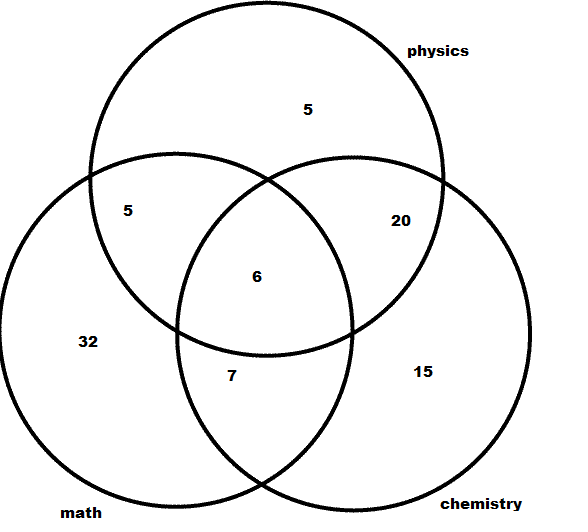# in a class ,  36 students offered physics , 48 offered chemistry and 50 offered mathematics . of these 13 are in both chm and maths 26 in phy and chm ,11 in maths and phy and 6 in al subjects. find how many students are there in the class . how many students offered only maths and how many students are exactly taking 2 of 3 subjects​

Dear Student,
You just need to draw the venn diagram for the given data.Steps to be followed while solving such problem,
1) number of students offering all three subject (PCM) should be entered first in the diagram.
2) now C and M =13, (13-6=5), P and C =26 (26-6=20), M and P = 11 (11-6=5).
3)P =36 (36-31), C= 48 (48- 33), M=50 , (50-18).
=
1) Total number of student in the class.
n=6+20+7+5+5+32+15=90 students.

2) students taking exact 2 subject,
=7+5+2=-14

Regards.

• -5Therefore No. of students in the class room =  a+b+c+d+e+f+g

= 90

No. of students who offerd only maths = g= 32

Students taking exactly 2 subjects = b+d+f

= 32

• 4
What are you looking for?